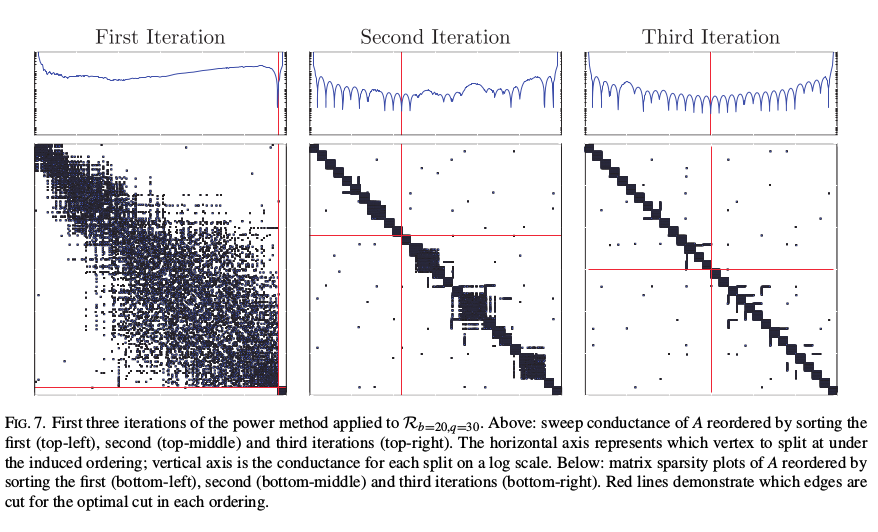# Graph Partitioning with Spectral Blends

### Abstract

Many common methods for data analysis rely on linear algebra. We provide new results connecting data analysis error to numerical accuracy in the context of spectral graph partitioning. We provide pointwise convergence guarantees so that spectral blends (linear combinations of eigenvectors) can be employed to solve data analysis problems with confidence in their accuracy. We apply this theory to an accessible model problem, the ring of cliques, by deriving the relevant eigenpairs and finding necessary and sufficient solver tolerances. Analysis of the ring of cliques provides an upper bound on eigensolver tolerances for graph partitioning problems. These results bridge the gap between linear algebra based data analysis methods and the convergence theory of iterative approximation methods. These results explain how the combinatorial structure of a problem can be recovered much faster than numerically accurate solutions to the associated linear algebra problem.

Type
Publication
Oxford Journal of Complex Networks
Date
Links